# Approximation theory

Jump to: navigation, search

The branch of mathematical analysis studying methods for approximating some mathematical objects by others and also studying questions related to the research and estimation of the error that arises here.

The main contents of approximation theory concerns the approximation of functions. Its foundations are laid by the work of P.L. Chebyshev (1854–1859) on best uniform approximation of functions by polynomials and by K. Weierstrass, who in 1885 established that in principle it is possible to approximate a continuous function on a finite interval by polynomials with arbitrary pre-given error. The development of approximation theory was to a large extent determined by the fundamental work of H. Lebesgue, Ch.J. de la Vallée-Poussin, S.N. Bernstein [S.N. Bernshtein], D. Jackson, J. Favard, A.N. Kolmogorov and S.M. Nikol'skii on the approximation of functions and function classes.

With the development of functional analysis, many problems in approximation theory were considered in the most general setting, e.g. as the approximation of elements of an arbitrary linear normed space. Three classes of problems arose here, corresponding more or less to the main chronological stages of development of research in approximation theory.

1) The approximation of a fixed elementby elements of a fixed set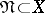. Ifis taken as the approximation measure, i.e. the best approximation ofby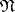, then, along with the investigation and estimation of, questions on the existence of an element of best approximation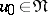(for which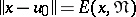), its uniqueness and characteristic features arise. Any operatormappingintogives rise to an approximation method with error. Ifis a linear manifold, linear operators are of particular importance. For sequences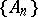of such operators, the question of the conditions of convergence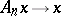for anyarises.

2) The approximation of a fixed set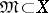by elements of another fixed set. The best approximation here is expressed bywhich gives the minimal possible error estimate when approximating an arbitraryby elements from. In concrete cases, the problem consists of estimating, or expressing exactly,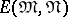by characteristic properties of the given sets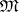and. If the approximation is established by an operator, the supremum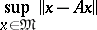is investigated, as well as (ifis a linear manifold)where the infimum is taken over all linear operatorsmappinginto. A linear operator realizing this infimum (if it exists) gives rise to a best linear method of approximation. The caseis of particular interest.

3) Best approximation of a fixed setby a given class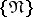of approximating sets in. It is assumed that inthere are, in a definite sense, "equally-expensive" classes, e.g. containing the same amount of elements or having the same dimension. The first case leads to the-entropy of(relative to), the second — to the problem of calculating the width of(in), in particular,(1)

and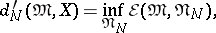(2)

where the infimum is taken over all subspacesinof fixed dimension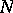(or over all possible translations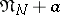of it). Thus in (1), (2) the problem is to determine the best (respectively the best linear) approximation tool of dimensionfor.